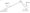# Engineering Mechanics Dec 2007

1. (a) Explain Virginian’s theorem.

(b) Solve any two parts:

(i) Two rollers of weight P and Q are connected by a flexib string AB. The rollers rests on two mutually perpendicul planes DE and EF as shown. Find the tension in the strir angle 0 that it makes with the horizontal when the system in equilibrium.

Fig;-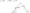(ii) A uniform beam AB of weight W = 100N . Rests on two r< supports C and D as shown if force of 250 N is applied tc end B find the range of the values of force F for which b< will remain in equilibrium

Fig:-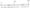(iii) Four forces acts on a plate as shown (a) find the resultafit these forces, (b) Locate the two points where the lin! action of resultant intersects the edge of the plate.

Fig:-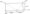Q.2.(a) What is shear force and bending moment and what relation between them.

(b) Solve any two parts :

(i) Determine the forces in each member of the truss shown.

(ii) Determine the forces in members BD and DE of the shown.

Fig:-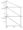(iii) Draw the shear and bending moment of diagram for the beam and loading shown.

Fig:-Q.3.(a) Find the relation between the tension of a belt and prove that Where T1 = Tension of tight side,

u=Coefficient of prism, T2 = Tension on loose side p — Angle of overlap. T1/T2=ei0

(b) Solve any two parts :

(i) Two identical planes AC and BC inclined 60° and 30° tc the horizontal meet at JC. A load of 1000N rests on the inclined plane BC and it tied by a rope passing over a pulley to a block weighing W Newton’s and resting on the plane AC as shown . If the coefficient of friction the block and plane AC is 0.02 find the least and the greatest value of W foi equilibrium of the system.

Fig:-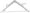(ii) Determine the force P required to start the movement of the wedge as shown. The angle friction for all surfaces in contact is 15*

Fig:-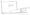(iii) Find the number of rope required to transmit 50KW. The maximum permissible tension in the rope is 1500 N. Velocity of the rope is 10m/sec and weright of the rope is 4N/m. Assume the angles of contact is 180* and pulley groove angle as 60*.

Q.4.(a) What is parallel axis theorem?

(b) Solve any two parts:-

(i) Determine the moment if inertia of the shaded area shown with the respect to each of the coordinate axes.

Fig;-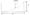(ii) Determine the product of inertia of the figure:-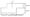(iii) Determine the moment if inertia of a right circular respect to (i) Longitudinal axis, (ii) an axis centurion of the cone and to the longitudinal axis.

Q.5.(a) What is principle of work energy ?

(b) Solve any two parts :

(i) Two blocks A and B are released from rest when they are 18m apart. The coefficient of friction under block A is 0.2 and that under lower block B is 0.4 .” In what time block A strikes block B and where w. = 100 N, w„ = 80 N

FIg:-(ii) A 3000N block starting from rest as shown slides down an incline after moving 2m it strikes a spring whose modules is 20N/mm. If the coefficient of friction between the block and the incline is 0.2 determine the maximum deformation of the spring and the maximum velocity of block.

Fig:-(iii) Determine the time required for the weights shown in fig. to attain a velocity of 9.81 m/sec. What is the tension in the chord ? Take = 0.2 for both planes. Assume pulls are frictionless.

Fig:-